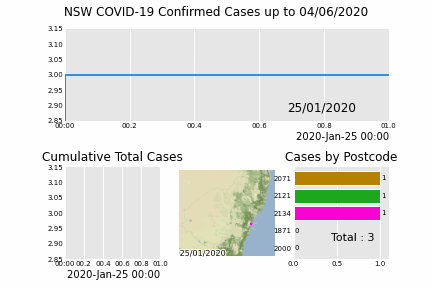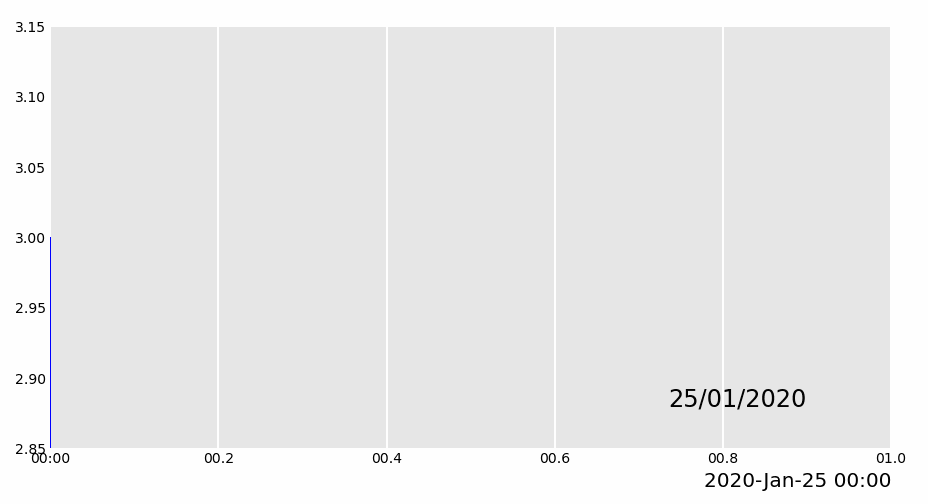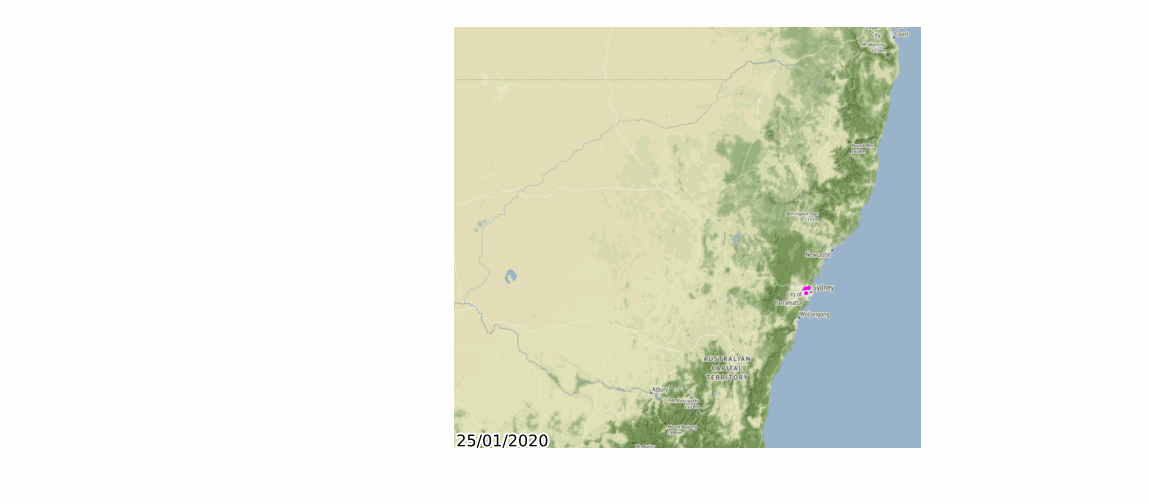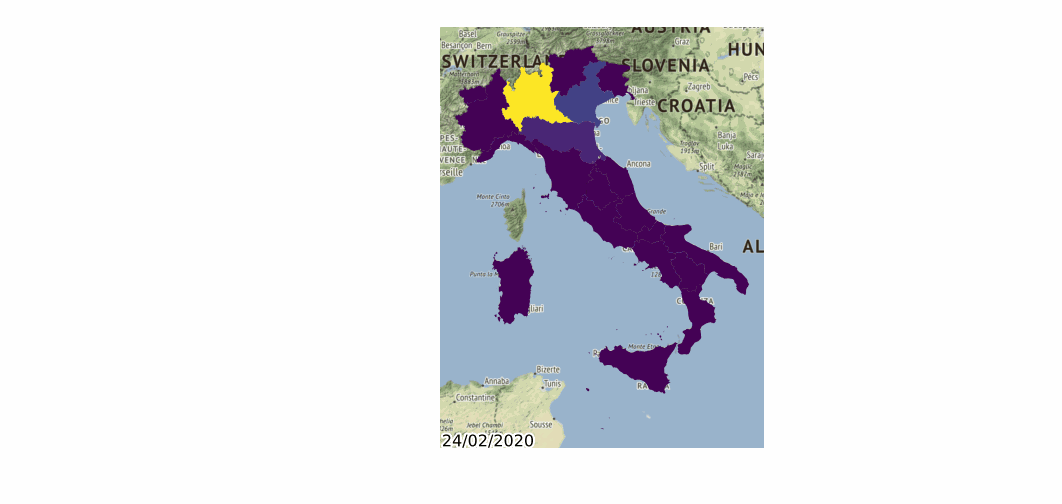# Geopandas and Pandas Alive

Posted on Fri 12 June 2020 in Python • 6 min read

Geopandas and Pandas_Alive

Following on from a previous post on making animated charts with `pandas_alive`, let's go into generating animated charts specifically for geospatial data with `geopandas`. Support for `geopandas` was introduced into `pandas_alive` in version 0.2.0, along with functionality to interface with `contextily` for enabling basemaps. The visualisation(s) we will make today, are initially was `pandas_alive` was created for!

When setting up `geopandas` & `pandas_alive` on Windows, the recommended set up is using Anaconda as `geopandas` requires GDAL, which is not a trivial process to set up on Windows. Luckily Anaconda distributes GDAL along with geopandas so we don't have to worry about it. We also need to install `descartes` (support for plotting polygons) and `contextily` for basemap support. These can be installed with:

• `descartes` : `conda install -c conda-forge descartes`
• `contextily` : `conda install -c conda-forge contextily`

`pandas_alive` also supports progress bars with `tqdm`, this can be installed via `conda install tqdm` and enabled using the enable_progress_bar=True keyword in `plot_animated()`

First off let's check out the end-result visualisation we'll be building today:Now let's get started, as always we begin by importing all the neccessary libraries.

In :
```import geopandas
import pandas as pd
import pandas_alive
import contextily
import matplotlib.pyplot as plt

import urllib.request, json
```

The data we wish to visualise is hosted through an API, so we will use `urllib` to load the json response and then find the dataset link (provided as a csv). Once we determine what the link is, we can use `pandas` to read the csv directly from the url. We also read in a dataset of matching geospatial co-ordinates to the postcodes.

In :
```with urllib.request.urlopen(
"https://data.nsw.gov.au/data/api/3/action/package_show?id=aefcde60-3b0c-4bc0-9af1-6fe652944ec2"
) as url:
data = json.loads(url.read().decode())

# Extract url to csv component
covid_nsw_data_url = data["result"]["resources"]["url"]

# Read csv from data API url
nsw_covid = pd.read_csv(covid_nsw_data_url)

# Source for postcode dataset https://www.matthewproctor.com/australian_postcodes
postcode_dataset = pd.read_csv("data/postcode-data.csv")

display(nsw_covid.head())
display(postcode_dataset.head())
```
notification_date postcode lhd_2010_code lhd_2010_name lga_code19 lga_name19
0 25/01/2020 2134.0 X700 Sydney 11300.0 Burwood (A)
1 25/01/2020 2121.0 X760 Northern Sydney 16260.0 Parramatta (C)
2 25/01/2020 2071.0 X760 Northern Sydney 14500.0 Ku-ring-gai (A)
3 27/01/2020 2033.0 X720 South Eastern Sydney 16550.0 Randwick (C)
4 1/03/2020 2077.0 X760 Northern Sydney 14000.0 Hornsby (A)
ID Postcode Locality State Longitude Latitude Category Type SA3 SA3 Name SA4 SA4 Name Status
0 458 1001 SYDNEY NSW 151.268071 -33.794883 LVR LVR 11703.0 Sydney Inner City 117.0 Sydney - City and Inner South Updated 25-Mar-2020 SA3
1 459 1002 SYDNEY NSW 151.268071 -33.794883 LVR LVR 11703.0 Sydney Inner City 117.0 Sydney - City and Inner South Updated 25-Mar-2020 SA3
2 460 1003 SYDNEY NSW 151.268071 -33.794883 LVR LVR 11703.0 Sydney Inner City 117.0 Sydney - City and Inner South Updated 25-Mar-2020 SA3
3 461 1004 SYDNEY NSW 151.268071 -33.794883 LVR LVR 11703.0 Sydney Inner City 117.0 Sydney - City and Inner South Updated 25-Mar-2020 SA3
4 462 1005 SYDNEY NSW 151.268071 -33.794883 LVR LVR 11703.0 Sydney Inner City 117.0 Sydney - City and Inner South Updated 25-Mar-2020 SA3

This data isn't in the format we need it to be, so let's do some preprocessing, in particular we:

• Fill in any gaps (with error value 9999)
• Convert the date string to a datetime object
• Groupby to get number of cases by date & postcode
• Unstack the multi-index that groupby returns
• Drop the unused column level
• Fill any missing values now with 0 cases (as these would be unprovided)
In :
```# Prepare data from NSW health dataset
nsw_covid = nsw_covid.fillna(9999)
nsw_covid["postcode"] = nsw_covid["postcode"].astype(int)

# Convert the date time string to a datetime object
nsw_covid['notification_date'] = pd.to_datetime(nsw_covid['notification_date'],dayfirst=True)

grouped_df = nsw_covid.groupby(["notification_date", "postcode"]).size()
grouped_df = pd.DataFrame(grouped_df).unstack()
grouped_df.columns = grouped_df.columns.droplevel().astype(str)

grouped_df = grouped_df.fillna(0)
grouped_df.index = pd.to_datetime(grouped_df.index)

cases_df = grouped_df

cases_df.to_csv('data/nsw-covid-cases-by-postcode.csv')
```

Now we can start by creating an area chart, and labelling any events in particular with vertical bars.

In :
```from datetime import datetime

bar_chart = cases_df.sum(axis=1).plot_animated(
filename='area-chart.gif',
kind='line',
label_events={
'Ruby Princess Disembark':datetime.strptime("19/03/2020", "%d/%m/%Y"),
'Lockdown':datetime.strptime("31/03/2020", "%d/%m/%Y")
},
fill_under_line_color="blue",
enable_progress_bar=True
)
```
```Generating LineChart, plotting ['0']
100%|██████████| 941/941 [17:54<00:00,  1.14s/it]
```Now it's time to prepare the dataset for our geospatial visualisations with `geopandas`. In particular:

• Drop any invalid longitudes / latitudes from our postcode dataset
• Drop any longitudes / latitudes that are 0
• Match the postcodes in each dataset to retrieve the equivalent longitude / latitude
• Remove the redundant/duplicated columns
• Package into a geopackage (ensure to keep the index column separate)
In :
```# Clean data in postcode dataset prior to matching

grouped_df = grouped_df.T
postcode_dataset = postcode_dataset[postcode_dataset['Longitude'].notna()]
postcode_dataset = postcode_dataset[postcode_dataset['Longitude'] != 0]
postcode_dataset = postcode_dataset[postcode_dataset['Latitude'].notna()]
postcode_dataset = postcode_dataset[postcode_dataset['Latitude'] != 0]
postcode_dataset['Postcode'] = postcode_dataset['Postcode'].astype(str)

# Build GeoDataFrame from Lat Long dataset and make map chart
grouped_df['Longitude'] = grouped_df.index.map(postcode_dataset.set_index('Postcode')['Longitude'].to_dict())
grouped_df['Latitude'] = grouped_df.index.map(postcode_dataset.set_index('Postcode')['Latitude'].to_dict())
gdf = geopandas.GeoDataFrame(
grouped_df, geometry=geopandas.points_from_xy(grouped_df.Longitude, grouped_df.Latitude),crs="EPSG:4326")
gdf = gdf.dropna()

# Prepare GeoDataFrame for writing to geopackage
gdf = gdf.drop(['Longitude','Latitude'],axis=1)
gdf.columns = gdf.columns.astype(str)
gdf['postcode'] = gdf.index
gdf.to_file("data/nsw-covid19-cases-by-postcode.gpkg", layer='nsw-postcode-covid', driver="GPKG")
```

Before we merge together all the charts, let's plot the prepared geospatial data on it's own.

In :
```# Prepare GeoDataFrame for plotting
gdf.index = gdf.postcode
gdf = gdf.drop('postcode',axis=1)
gdf = gdf.to_crs("EPSG:3857") #Web Mercator

map_chart = gdf.plot_animated(filename='map-chart.gif',title="Cases by Location",basemap_format={'source':contextily.providers.Stamen.Terrain},cmap='cool')
```Finally let's merge all these charts together into a single chart!

In :
```grouped_df = pd.read_csv('data/nsw-covid-cases-by-postcode.csv', index_col=0, parse_dates=)

line_chart = (
grouped_df.sum(axis=1)
.cumsum()
.fillna(0)
.plot_animated(kind="line", period_label=False, title="Cumulative Total Cases")
)

def current_total(values):
total = values.sum()
s = f'Total : {int(total)}'
return {'x': .85, 'y': .2, 's': s, 'ha': 'right', 'size': 11}

race_chart = grouped_df.cumsum().plot_animated(
n_visible=5, title="Cases by Postcode", period_label=False,period_summary_func=current_total
)

import time

timestr = time.strftime("%d/%m/%Y")

plots = [bar_chart, line_chart, map_chart, race_chart]

from matplotlib import rcParams

rcParams.update({"figure.autolayout": False})

figs = plt.figure()
gs = figs.add_gridspec(2, 3, hspace=0.5)
f3_ax1 = figs.add_subplot(gs[0, :])
f3_ax1.set_title(bar_chart.title)
bar_chart.ax = f3_ax1

f3_ax2 = figs.add_subplot(gs[1, 0])
f3_ax2.set_title(line_chart.title)
line_chart.ax = f3_ax2

f3_ax3 = figs.add_subplot(gs[1, 1])
f3_ax3.set_title(map_chart.title)
map_chart.ax = f3_ax3

f3_ax4 = figs.add_subplot(gs[1, 2])
f3_ax4.set_title(race_chart.title)
race_chart.ax = f3_ax4

timestr = cases_df.index.max().strftime("%d/%m/%Y")
figs.suptitle(f"NSW COVID-19 Confirmed Cases up to {timestr}")

pandas_alive.animate_multiple_plots(
'nsw-covid.gif',
plots,
figs
)
```
```Generating LineChart, plotting ['0']
Generating BarChartRace, plotting ['1871', '2000', '2007', '2008', '2009', '2010', '2011', '2015', '2016', '2017', '2018', '2019', '2020', '2021', '2022', '2023', '2024', '2025', '2026', '2027', '2028', '2029', '2030', '2031', '2032', '2033', '2034', '2035', '2036', '2037', '2038', '2039', '2040', '2041', '2042', '2043', '2044', '2045', '2046', '2047', '2048', '2049', '2050', '2060', '2061', '2062', '2063', '2064', '2065', '2066', '2067', '2068', '2069', '2070', '2071', '2072', '2073', '2074', '2075', '2076', '2077', '2079', '2080', '2081', '2084', '2085', '2086', '2087', '2088', '2089', '2090', '2091', '2092', '2093', '2094', '2095', '2096', '2097', '2099', '2100', '2101', '2102', '2103', '2104', '2106', '2107', '2108', '2110', '2111', '2112', '2113', '2114', '2115', '2116', '2117', '2118', '2119', '2120', '2121', '2122', '2125', '2126', '2127', '2128', '2130', '2131', '2132', '2134', '2135', '2137', '2138', '2140', '2141', '2142', '2144', '2145', '2147', '2148', '2150', '2151', '2152', '2153', '2154', '2155', '2156', '2158', '2159', '2160', '2161', '2162', '2163', '2164', '2165', '2166', '2168', '2170', '2171', '2172', '2173', '2176', '2177', '2178', '2179', '2190', '2191', '2192', '2193', '2194', '2195', '2196', '2197', '2198', '2199', '2200', '2203', '2204', '2205', '2206', '2207', '2208', '2209', '2210', '2211', '2212', '2213', '2216', '2217', '2218', '2219', '2220', '2221', '2223', '2224', '2225', '2226', '2227', '2228', '2229', '2230', '2231', '2232', '2233', '2234', '2250', '2251', '2256', '2257', '2258', '2259', '2260', '2261', '2262', '2263', '2264', '2265', '2278', '2280', '2281', '2282', '2283', '2284', '2285', '2286', '2287', '2289', '2290', '2291', '2292', '2297', '2298', '2299', '2300', '2303', '2304', '2305', '2306', '2315', '2316', '2317', '2318', '2319', '2320', '2321', '2322', '2323', '2324', '2325', '2327', '2330', '2333', '2334', '2335', '2337', '2340', '2343', '2345', '2350', '2357', '2358', '2360', '2371', '2372', '2380', '2400', '2420', '2421', '2422', '2423', '2425', '2427', '2428', '2430', '2431', '2439', '2440', '2443', '2444', '2445', '2446', '2447', '2448', '2450', '2452', '2454', '2456', '2460', '2463', '2464', '2465', '2470', '2476', '2477', '2478', '2479', '2480', '2481', '2482', '2483', '2484', '2485', '2486', '2487', '2490', '2500', '2505', '2506', '2508', '2515', '2516', '2517', '2518', '2519', '2525', '2526', '2527', '2528', '2529', '2530', '2533', '2535', '2536', '2537', '2539', '2540', '2541', '2546', '2548', '2550', '2557', '2558', '2560', '2564', '2565', '2566', '2567', '2568', '2569', '2570', '2571', '2575', '2576', '2577', '2578', '2580', '2581', '2582', '2583', '2586', '2590', '2619', '2620', '2621', '2627', '2628', '2630', '2631', '2640', '2641', '2642', '2643', '2644', '2646', '2647', '2650', '2660', '2680', '2700', '2711', '2713', '2714', '2716', '2722', '2745', '2747', '2748', '2749', '2750', '2752', '2753', '2754', '2756', '2757', '2758', '2759', '2760', '2761', '2763', '2765', '2766', '2767', '2768', '2769', '2770', '2773', '2774', '2777', '2779', '2780', '2782', '2783', '2785', '2786', '2790', '2795', '2799', '2800', '2810', '2820', '2821', '2824', '2830', '2843', '2849', '2850', '2865', '2866', '2870', '2880', '9999']
```Pandas_Alive also supports animating polygon GeoDataFrames!

In [ ]:
```import geopandas
import pandas_alive
import contextily

gdf = geopandas.read_file('data/italy-covid-region.gpkg')
gdf.index = gdf.region
gdf = gdf.drop('region',axis=1)

map_chart = gdf.plot_animated(filename='examples/example-geo-polygon-chart.gif',basemap_format={'source':contextily.providers.Stamen.Terrain})
```In [ ]:
```
```